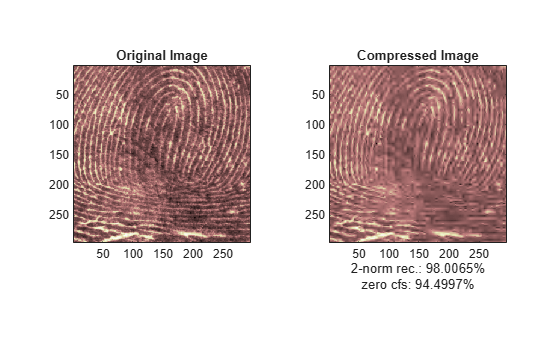# wdcbm2

Thresholds for wavelet 2-D using Birgé-Massart strategy

## Syntax

``[thr,nkeep] = wdcbm2(C,S,alpha,M)``

## Description

example

````[thr,nkeep] = wdcbm2(C,S,alpha,M)` returns level-dependent thresholds `thr` and numbers of coefficients to be kept `nkeep`, for denoising or compressing an image. `wdcbm2` uses a wavelet coefficients selection rule based on the Birgé-Massart strategy to obtain the thresholds.`[C,S]` is the wavelet decomposition structure of the image to be denoised or compressed, at level ```N = size(S,1)-2```. `alpha` and `M` are real numbers greater than 1.`wdcbm2(C,S,alpha)` is equivalent to `wdcbm2(C,S,alpha,prod(S(1,:)))`.```

## Examples

collapse all

```load detfingr nbc = size(map,1);```

Obtain the wavelet decomposition of the image at level 3 using the `sym4` wavelet.

```wname = "sym4"; lev = 3; [c,s] = wavedec2(X,lev,wname);```

Use `wdcbm2` to select level-dependent thresholds for image compression. Use the suggested parameters.

```alpha = 1.5; m = 2.7*prod(s(1,:)); [thr,nkeep] = wdcbm2(c,s,alpha,m)```
```thr = 3×3 21.4814 46.8354 40.7907 21.4814 46.8354 40.7907 21.4814 46.8354 40.7907 ```
```nkeep = 1×3 624 961 1765 ```

Use `wdencmp2` for compressing the image using the thresholds. Use hard thresholding.

```[xd,cxd,sxd,perf0,perfl2] = ... wdencmp("lvd",c,s,wname,lev,thr,"h");```

Plot the original and compressed images.

```colormap(pink(nbc)) subplot(2,2,1) image(wcodemat(X,nbc)) title("Original Image") subplot(2,2,2) image(wcodemat(xd,nbc)) title("Compressed Image") xlab1 = ['2-norm rec.: ',num2str(perfl2)]; xlab2 = [' % -- zero cfs: ',num2str(perf0), ' %']; xlabel([xlab1 xlab2]);```## Input Arguments

collapse all

Wavelet decomposition of the image to be denoised or compressed, specified as a real-valued vector. The vector `C` contains the approximation and detail coefficients organized by level. The bookkeeping matrix `S` is used to parse `C`. See `wavedec2`.

Data Types: `double`

Bookkeeping matrix, specified as an integer-valued matrix. The matrix `S` contains the dimensions of the wavelet coefficients by level and is used to parse the wavelet decomposition vector `C`. See `wavedec2`.

Data Types: `double`

Sparsity parameter to use in the Birgé-Massart strategy, specified as a real-valued scalar greater than 1. Typically, ```alpha = 1.5``` for compression and ```alpha = 3``` for denoising. For more information, see Wavelet Coefficients Selection.

Data Types: `double`

Factor to use in the Birgé-Massart strategy, specified as a real-valued scalar greater than 1. The default value is `prod(S(1,:))`, the length of the coarsest approximation coefficients. Recommended values for `M` are from `prod(S(1,:))` to `6*prod(S(1,:))`. For more information, see Wavelet Coefficients Selection.

Data Types: `double`

## Output Arguments

collapse all

Level-dependent thresholds, returned as a 3-by-`N` matrix, where `N = size(S,1)-2`. `thr(:,i)` contains the thresholds in the three orientations: horizontal, diagonal, and vertical, for level i. The thresholds are obtained using a wavelet coefficients selection rule based on the Birgé-Massart strategy. For more information, see Wavelet Coefficients Selection.

Number of coefficients to be kept at each level, returned as a vector of length `N`, where ```N = size(S,1)-2```. `nkeep(i)` contains the number of coefficients to be kept at level i.

Data Types: `double`

collapse all

### Wavelet Coefficients Selection

Thresholds are obtained using a wavelet coefficients selection rule based on the Birgé-Massart strategy. The values ```N = prod(S(1,:))```, `M` and `alpha` define the strategy.

• At level `N+1` (and coarser levels), everything is kept.

• For level i from 1 to `N`, the ni largest coefficients are kept, where ```ni = M / (N+2-i)alpha```.

The default value of ```M = prod(S(1,:))``` corresponds to the formula ```nN+1 = M / (N+2-(N+1))alpha = M```.

 Birgé, Lucien, and Pascal Massart. “From Model Selection to Adaptive Estimation.” In Festschrift for Lucien Le Cam: Research Papers in Probability and Statistics, edited by David Pollard, Erik Torgersen, and Grace L. Yang, 55–87. New York, NY: Springer, 1997. https://doi.org/10.1007/978-1-4612-1880-7_4.